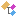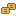﻿ Nevron.SmartShapes Namespace
Nevron .NET Vision
Inheritance Hierarchy
In This Topic
Nevron.SmartShapes Namespace
In This Topic
Classes
ClassDescriptionSummary description for N1DSmartShapeFactory.Summary description for N2DSmartShapeFactory.The NArcToRow class represents a path command row, which plots an arc from the previous command (X, Y) cells to this row (X, Y) cells. The A cell defines the arc bow (the distance from the arc's midpoint to the midpoint of its chord)The NBezierCurveToRow class represents a path command row, which plots a bezier curve from the previous command (X, Y) cells to this row (X, Y) cells. The (A, B) cells define the first control point. The (C, D) cells define the second control point.The NChangePathCommandTypeVerb verb is used for the visual changing of the path command row typeThe NCircularArcToRow class represents a path command row, which plots a circular arc from the previous command (X, Y) cells to this row (X, Y) cells. The (A, B) cells define the circle control point.The NControlBehaviorFormulaCell is a formula cell, which has a strong type of Int32 and provides options from the ControlBehavior enumerationThe NControlsRow class represents a formula row, which is used for the formula definition of a control inside a controls sectionsThe NControlsSection class represents a formula section, which is used to expose a set of control points, which can be used for the interactive modification of the smart shape geometryThe NEllipticalArcToRow class represents a path command row, which plots an elliptical arc from the previous command (X, Y) cells to this row (X, Y) cells. The (A, B) cells define the ellipse control point. The C parameter defines the angle of the ellipse major axis. The D parameter defines the ratio between the major and minor ellipse axes.The NEndPointsSection class represents a formula section, which is used to expose formula based start and end pointsThe NGeometrySection class represents a formula section, which is used for the formula definition of a graphics path inside a shape sheet.The NInsertSectionsVerb verb is used for the visual insertion of path sheet sectionsThe NLineToRow class represents a path command row, which plots a line from the previous command (X, Y) cells to this row (X, Y) cells.The NMoveToRow class represents a path command row, which moves the plotter to the location identified by its (X, Y) cells.The NPathCommandRow class represents a formula row, which is used for the formula definition of a single path command inside a formula figure. It serves as base class for more specific path commands.The NRemoveSectionsVerb verb is used for the visual removal of path sheet sectionsThe NScratchRow class represents a formula row, which is used for the formula definition of a scratch inside a scratch sectionsThe NScratchSection class represents a formula section, which is used for the intermediate calculation of commonly used expressions in the path figure formula definitionsThe NShapeSheet class represents a formula sheet, which is used for the formula definition of a smart shapeComparer used for the default sorting of the shape sheet sectionsThe NSmartGroup class is a smart shape, which can contain other smart shapesThe NSmartShape class represents a shape, much of which content is defined by an underlying shape sheet.The NSmartShapeCollection class is a strongly typed collection, containing NSmartShape instancesThe NSmartShapeDocument class is used for the serialization of smart shapesThe NSmartShapeDocumentSerializer class facilitates the persistency of smart shape documents.The base of all shape factoriesThe NSmartShapesCategory class represents a collection of smart shapes, which can be passed to the shape editorThe NSmartShapeSerializer class facilitates the persistency of smart shapes.The NTransformSection class represents a formula section, which is used for the formula definition of a transformation, which transforms the smart shape to the coordinate system of its parentThe NUserDefinedCellsSection class represents a formula section, which inserts an area of nameable cells for entering formulas and constants.
Enumerations
EnumerationDescriptionEnumerates the possible ways in which controls X and Y coordinates can behaveEnumerates the 2D smart shapes supported by the N1DSmartShapeFactory class.Enumerates the 2D smart shapes supported by the N2DSmartShapeFactory class.